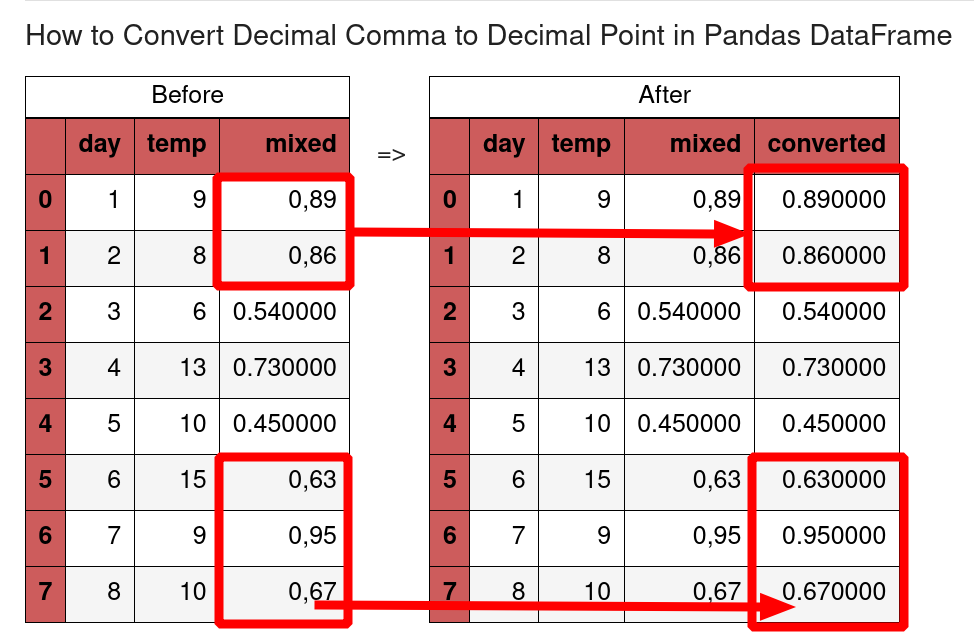In this quick tutorial, we're going to convert decimal comma to decimal point in Pandas DataFrame and vice versa. It will also show how to remove decimals from strings in Pandas columns.

Different people in the world are using different decimal separator like:

• decimal point - more often
• decimal comma - in the Francophone area

## Setup

Let's work with the following DataFrame:

``````import pandas as pd

df = pd.DataFrame(data={'day': [1, 2, 3, 4, 5, 6, 7, 8],
'temp': [9, 8, 6, 13, 10, 15, 9, 10],
'humidity': [0.89, 0.86, 0.54, 0.73, 0.45, 0.63, 0.95, 0.67],
'humidity_eu': ['0,89', '0,86', '0,54', '0,73', '0,45', '0,63', '0,95', '0,67']})
``````

We have two columns with float data:

• decimal comma
• decimal point
day temp humidity humidity_eu
0 1 9 0.89 0,89
1 2 8 0.86 0,86
2 3 6 0.54 0,54
3 4 13 0.73 0,73
4 5 10 0.45 0,45

## 1: read_csv - decimal point vs comma

Let's start with the optimal solution - convert decimal comma to decimal point while reading CSV file in Pandas.

Method `read_csv()` has parameter three parameters that can help:

• `decimal` - the decimal sign used in the CSV file
• `delimiter` - separator for the CSV file (tab, semi-colon etc)
• `thousands` - what is the symbol for thousands - if any

To use them we can do:

``````df = pd.read_csv('file.csv', delimiter=";", decimal=",", thousands="`")
``````

This will ensure that the correct decimal symbol is used for the DataFrame.

## 2: Convert comma to point

If the DataFrame contains values with comma then we can convert them by `.str.replace()`:

``````df['humidity_eu'].str.replace(',', '.').astype(float)
``````

result is:

``````0    0.89
1    0.86
2    0.54
3    0.73
4    0.45
5    0.63
6    0.95
7    0.67
Name: humidity_eu, dtype: float64
``````

## 3: Mixed decimal data - point and comma

What can we do in case of mixed data in a given column? For this example we can use: list comprehensions and `pd.to_numeric()`.

This can help us to identify the problematic values and keep the rest the same.

For example we can do:

``````s = pd.Series(['0,89', '0,86', 0.54, 0.73, 0.45, '0,63', '0,95', '0,67'])
mix = [x.replace(',', '.') if type(x) == str else x for x in s]
``````

to replace the comma in all string records:

``````['0.89', '0.86', 0.54, 0.73, 0.45, '0.63', '0.95', '0.67']
``````

Then we can convert the Series by:

``````pd.to_numeric(mix)
``````
``````array([0.89, 0.86, 0.54, 0.73, 0.45, 0.63, 0.95, 0.67])
``````

## 4: Detect decimal comma in mixed column

To detect which are the problematic values we can use:

``````s[pd.to_numeric(s, errors='coerce').isna() ]
``````

the result of `to_numeric` is:

``````array([ nan,  nan, 0.54, 0.73, 0.45,  nan,  nan,  nan])
``````

while the final result is showing all values with decimal comma:

``````0    0,89
1    0,86
5    0,63
6    0,95
7    0,67
dtype: object
``````

## 5: to_csv - decimal point vs comma

Finally if we like to write CSV file by method `to_csv` we can use parameters:

• `decimal`
• `sep`

to control the decimal symbol.

To convert CSV values from decimal comma to decimal point with Python and Pandas we can do :

``````df = pd.read_csv("file.csv", decimal=",")
df.to_csv("test2.csv", sep=',', decimal='.')
``````## ValueError: could not convert string to float: '0,89'

The error: "ValueError: could not convert string to float: '0,89'" is raised when we try to parse decimal comma to float.

The error is given by method `s.astype(float)`:

``````s = pd.Series(['0,89', '0,86', 0.54, 0.73, 0.45, '0,63', '0,95', '0,67'])
s.astype(float)
``````

## ValueError: Unable to parse string "0,89" at position 0

The error is the result of the `pd.to_numeric(s)` method - when a decimal comma is present in the input values.

``````s = pd.Series(['0,89', '0,86', 0.54, 0.73, 0.45, '0,63', '0,95', '0,67'])
pd.to_numeric(s)
``````

result:

``````ValueError: Unable to parse string "0,89" at position 0
``````

## Conclusion

In this article we saw how to replace, change and convert decimal symbols in Pandas. We saw how to detect problematic values in mixed columns - which have decimal commas and points simultaneously.

Typical errors were explained.

For further reference you can check also: